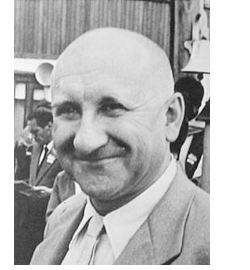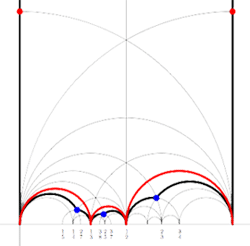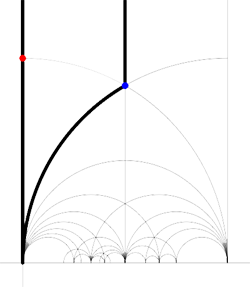In older NeverEndingBooks-posts (and here) proofs were given that the modular group $\Gamma = PSL_2(\mathbb{Z})$ is the group free product $C_2 \ast C_3$, so let’s just skim over details here. First one observes that $\Gamma$ is generated by (the images of) the invertible 2×2 matrices

$U= \begin{bmatrix} 0 & -1 \\\ 1 & 0 \end{bmatrix}$ and $V= \begin{bmatrix} 0 & 1 \\\ -1 & 1 \end{bmatrix}$

A way to see this is to consider X=U.V and Y=V.U and notice that multiplying with powers of X adds multiples of the second row to the first (multiply on the left) or multiples of the first column to the second (multiply on the right) and the other cases are handled by taking multiples with powers of Y. Use this together with the fact that matrices in $GL_2(\mathbb{Z})$ have their rows and columns made of coprime numbers to get any such matrix by multiplication on the left or right by powers of X and Y into the form

$\begin{bmatrix} \pm 1 & 0 \\\ 0 & \pm 1 \end{bmatrix}$ and because $U^2=V^3=\begin{bmatrix} -1 & 0 \\\ 0 & -1 \end{bmatrix}$

we see that $\Gamma$ is an epimorphic image of $C_2 \ast C_3$. To prove isomorphism one can use the elegant argument due to Roger Alperin considering the action of the Moebius transformations $u(z) = -\frac{1}{z}$ and $v(z) = \frac{1}{1-z}$ (with $v^{-1}(z) = 1-\frac{1}{z}$) induced by the generators U and V on the sets $\mathcal{P}$ and $\mathcal{N}$ of all positive (resp. negative) irrational real numbers. Observe that

$u(\mathcal{P}) \subset \mathcal{N}$ and $v^{\pm}(\mathcal{N}) \subset \mathcal{P}$

Hence, if $w$ is a word in $u$ and $v^{\pm}$ of off length we either have $w(\mathcal{P}) \subset \mathcal{N}$ or $w(\mathcal{N}) \subset \mathcal{P}$ so $w$ can never be the identity. If the length is even we can conjugate $w$ such that it starts with $v^{\pm}$. If it starts with $v$ then $w(\mathcal{P}) \subset v(\mathcal{N})$ is a subset of positive rationals less than 1 whereas if it starts with $v^{-1}$ then $w(\mathcal{P}) \subset v^{-1}(\mathcal{N})$ is a subset of positive rationals greater than 1, so again it cannot be the identity. Done!By a result of Aleksandr Kurosh it follows that every modular subgroup is the group free product op copies of $C_2, C_3$ or $C_{\infty}$ and we would like to determine the free generators explicitly for a cofinite subgroup starting from its associated Farey code associated to a special polygon corresponding to the subgroup.

To every even interval $$\xymatrix{x_i = \frac{a_i}{b_i} \ar@{-}[r]_{\circ} & x_{i+1}= \frac{a_{i+1}}{b_{i+1}}}$$ in the Farey code one associates the generator of a $C_2$ component

$A_i = \begin{bmatrix} a_{i+1}b_{i+1}+ a_ib_i & -a_i^2-a_{i+1}^2 \\\ b_i^2+b_{i+1}^2 & -a_{i+1}b_{i+1}-a_ib_i \end{bmatrix}$

to every odd interval $$\xymatrix{x_i = \frac{a_i}{b_i} \ar@{-}[r]_{\bullet} & x_{i+1} = \frac{a_{i+1}}{b_{i+1}}}$$ in the Farey code we associate the generator of a $C_3$ component

$B_i = \begin{bmatrix} a_{i+1}b_{i+1}+a_ib_{i+1}+a_ib_i & -a_i^2-a_ia_{i+1}-a_{i+1}^2 \\\ b_i^2+b_ib_{i+1} + b_{i+1}^2 & -a_{i+1}b_{i+1} – a_{i+1}b_i – a_i b_i \end{bmatrix}$

and finally, to every pair of free intervals $$\xymatrix{x_k \ar@{-}[r]_{a} & x_{k+1}} \ldots \xymatrix{x_l \ar@{-}[r]_{a} & x_{l+1}}$$ we associate the generator of a $C_{\infty}$ component

$C_{k,l} = \begin{bmatrix} a_l & -a_{l+1} \\\ b_l & – b_{l+1} \end{bmatrix} \begin{bmatrix} a_{k+1} & a_k \\\ b_{k+1} & b_k \end{bmatrix}^{-1}$Kulkarni’s result states that these matrices are free generators of the cofiniite modular subgroup determined by the Farey code. For example, for the M(12) special polygon on the left (bounded by the thick black geodesics), the Farey-code for this Mathieu polygon is

$$\xymatrix{\infty \ar@{-}[r]_{1} & 0 \ar@{-}[r]_{\bullet} & \frac{1}{3} \ar@{-}[r]_{\bullet} & \frac{1}{2} \ar@{-}[r]_{\bullet} & 1 \ar@{-}[r]_{1} & \infty}$$

Therefore, the structure of the subgroup must be $C_{\infty} \ast C_3 \ast C_3 \ast C_3$ with the generator of the infinite factor being

$\begin{bmatrix} -1 & 1 \\\ -1 & 0 \end{bmatrix}$ and those of the cyclic factors of order three$\begin{bmatrix} 3 & -1 \\\ 13 & -4 \end{bmatrix}, \begin{bmatrix} 7 & -3 \\\ 19 & 8 \end{bmatrix}$ and $\begin{bmatrix} 4 & -3 \\\ 7 & -5 \end{bmatrix}$

This approach also gives another proof of the fact that $\Gamma = C_2 \ast C_3$ because the Farey code to the subgroup of index 1 is $$\xymatrix{\infty \ar@{-}[r]_{\circ} & 0 \ar@{-}[r]_{\bullet} & \infty}$$ corresponding to the fundamental domain on the left. This finishes (for now) this thread on Kulkarni’s paper (or rather, part of it). On the Lost? page I will try to list threads in a logical ordering when they materialize.

Reference

Ravi S. Kulkarni, “An arithmetic-geometric method in the study of the subgroups of the modular group”, Amer. J. Math 113 (1991) 1053-1133

Published in featured

## One Comment

This site uses Akismet to reduce spam. Learn how your comment data is processed.